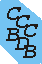Computational Chemistry Comparison and Benchmark DataBase Release 22 (May 2022) Standard Reference Database 101 National Institute of Standards and Technology Home All data for one species Geometry Experimental Calculated Comparisons Bad Calculations Tutorials and Explanations Vibrations Experimental Calculated Scale factors Reactions Entropies Ions List Ions Energy Electron Affinity Proton Affinity Ionization changes point group Experimental One molecule all properties One property a few molecules Geometry Vibrations Energy Electrostatics Reference Data Calculated Energy Optimized Reaction Internal Rotation Orbital Nuclear repulsion energy Correlation Ion Excited State Basis Set Extrapolation Geometry Vibrations Frequencies Zero point energy (ZPE) Scale Factors Bad Calculations Electrostatics Charges Dipole Quadrupole Polarizability Spin Entropy and Heat Capacity Reaction Lookup by property Comparisons Geometry Vibrations Energy Entropy Electrostatics Ion Resources Info on Results Calculations Done Basis functions used I/O files Glossary Conversion Forms Links NIST Links External links Thermochemistry Tutorials Vibrations Entropy Energy Electrostatics Geometry Cost Bad Calculations FAQ Help Units Choose Units Explanations Credits Just show me Summary Using List Recent molecules Molecules Geometry Vibrations Energy Similar molecules Ions, Dipoles, etc. Index of CCCBDB Feedback You are here: Calculated > Vibrations > Bad Calculations > Bad vib. Calc. vs exp. OR Resources > Bad Calculations > Vibrations > Bad vib. Calc. vs exp.

19 02 11 15 45

Calculated frequencies that differ from experiment by more than 400 cm-1 or have a ratio with experiment outside of 0.7 to 1.2 are listed

Calculated vibrational frequencies ("theory" in table) are calculated at MP3/SDD
Calculated values were scaled by 1.

Species Name mode int rot Symmetry Experiment Theory difference ratio
SO2 Sulfur dioxide 3 B2 1362 1131 -231 1.205
NH3 Ammonia 2 torsion A1 950 722 -228 1.316
Cl2 Chlorine diatomic 1 Σg 554 436 -118 1.271
NO Nitric oxide 1 Σ 1876 3711 1835 0.505
BF Boron monofluoride 1 Σ 1379 1141 -237 1.208